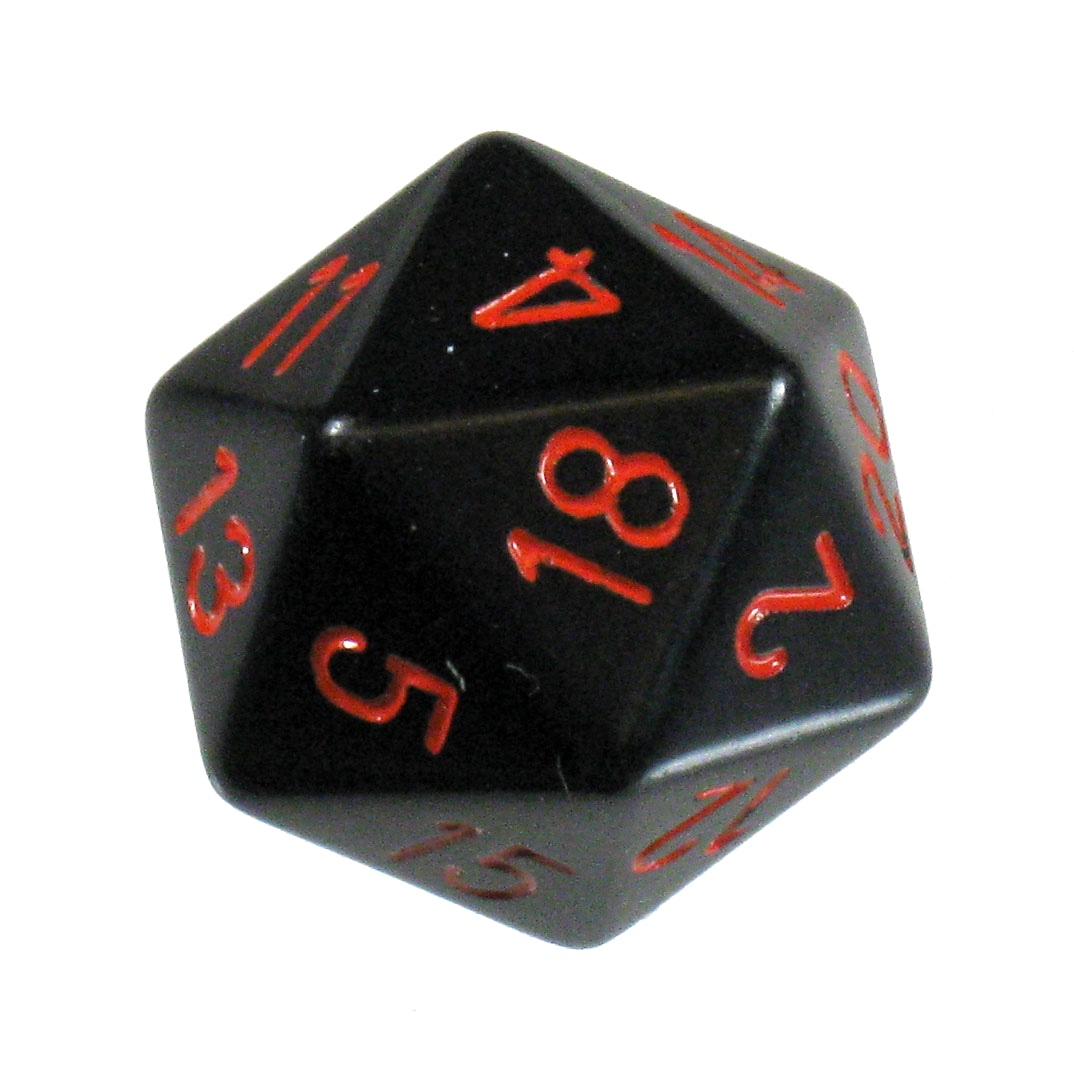Puzzles abound in the games the Varsity Math crew likes to play.### Different Dice

Nina is playing games with some of her friends when she notices that Rafa has an unusually-marked die. She asks him about it, and he says “Oh yes, that die is an icosahedron, as 20-sided dice usually are. But each face is marked with a positive integer so that the sum of the faces is equal to the answer to the Simultaneous Remainders problem, and so that the number of pairs of faces that share a vertex and show the same number is minimized. Neat, huh?”

What is the probability of rolling a 1 on Rafa’s die?

### No-Dispute Knights

Jorge is fascinated with the move of the chess knight: it attacks any square that differs from its current position either by two columns and one row, or by one column and two rows, no matter what other pieces are present. (And note that a knight is not considered to “attack” the square that it is currently on.) Sometimes Jorge thinks he’d like to play a version of chess that only used knights.

What is the maximum number of knights that can be placed in the central 4-by-4 region of a standard 8-by-8 chessboard so that no square on the chessboard is attacked by more than one knight (regardless of whether the attacked square is occupied)?

## Solution to Week 33

Subtle Sequences. If you simply try to calculate `a_2,b_2,a_3,b_3`, etc., to try to find a pattern, you may see what’s going on, but it is a lot of algebraic work. The best way to find the solution to this problem is to note the analogy between the rules defining the sequences and the angle sum formulas for sin and cos: `cos(alpha+beta) = cos alpha cos beta − sin alpha sin beta` and `sin(alpha + beta) = sin alpha cos beta + cos alpha sin beta`. In other words, if `a_1` were equal to `cos theta`, `b_1` equal to `sin theta`, `a_n` equal to `cos n theta`, and `b_n` equal to `sin n theta`, then `a_{n+1} = a_na_1 – b_nb_1 = cos n theta cos theta − sin n theta sin theta = cos(n theta + theta) = cos((n+1)theta)` and `b_{n+1} = b_na_1 + a_nb_1 = sin n theta cos theta + cos n theta sin theta = sin(n theta + theta) = sin((n+1)theta)`. Therefore, by induction, if `a_1=cos theta` and `b_1=sin theta`, then for all `n`, `a_n = cos n theta` and `b_n = sin n theta`.

But are `a_1` and `b_1` the cos and sin of some angle? Well, `a_1^2 + b_1^2 = (6+2sqrt5)//16 + (5-sqrt5)//8 = (6+2sqrt5)//16 + (10-2sqrt5)//16 = 16//16 = 1`, so yes, `(a_1,b_1)` lies on the unit circle in the plane, and so `a_1` and `b_1` must be the cos and sin of some angle. How to find out what angle? Four possible methods come to mind: (A) Use a calculator; (B) plot the point, guess what seems like a likely angle, and check your guess; (C) go ahead and calculate the first several `a_n` and `b_n` even though it’s a lot of algebraic work and discover to your pleasant surprise that `a_5` is -1 and `b_5` is 0; or (D) recognize `a_1` as `cos pi//5` from your extended special angle table. We’re going to assume you used method (D), since Coach Newton says folks on Varsity Math should know their special angles.

That means that `a_n = cos n pi//5`, so `a_{2016} = cos 2016pi//5 = cos 6pi//5`, which is the cos of the point exactly halfway around the circle from `(a_1,b_1)`, so `a_{2016}` is equal to `-a_1 = -(1+sqrt5)//4`.

## Recent Weeks

Links to all of the puzzles and solutions are on the Complete Varsity Math page.

Come back next week for answers and more puzzles.

[asciimathsf]